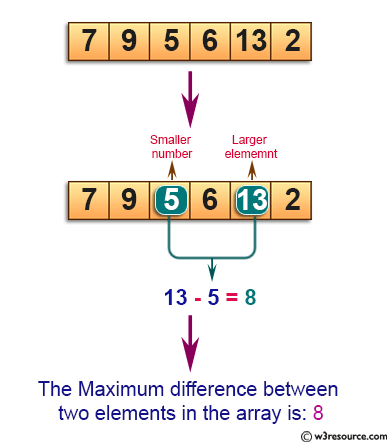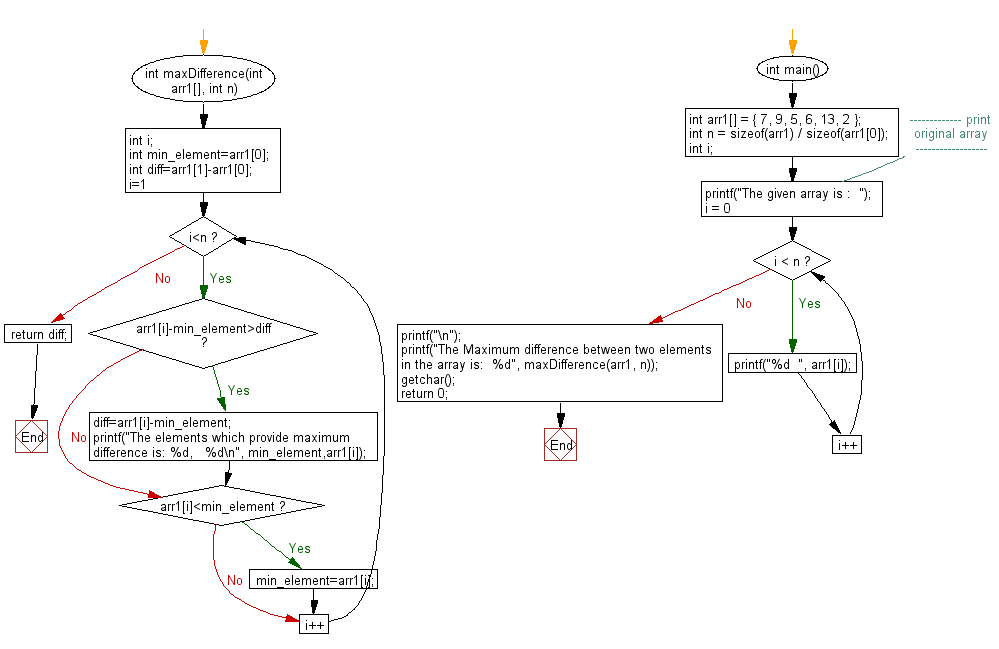﻿ C exercises: Find out the maximum difference between any two elements - w3resource# C Exercises: Find out the maximum difference between any two elements

## C Array: Exercise-69 with Solution

Write a program in C to find out the maximum difference between any two elements such that larger element appears after the smaller number.

Pictorial Presentation:Sample Solution:

C Code:

``````#include <stdio.h>

int maxDifference(int arr1[], int n)
{
int i;
int min_element=arr1;
int diff=arr1-arr1;
for(i=1;i<n;i++)
{
if(arr1[i]-min_element>diff)
{
diff=arr1[i]-min_element;
printf("The elements which provide maximum difference is: %d,   %d\n", min_element,arr1[i]);

}
if(arr1[i]<min_element)
min_element=arr1[i];
}
return diff;
}

int main()
{
int arr1[] =  { 7, 9, 5, 6, 13, 2 };
int n = sizeof(arr1) / sizeof(arr1);
int i;
//------------- print original array ------------------
printf("The given array is :  ");
for(i = 0; i < n; i++)
{
printf("%d  ", arr1[i]);
}
printf("\n");
//------------------------------------------------------

printf("The Maximum difference between two elements in the array is:  %d",  maxDifference(arr1, n));
getchar();
return 0;
}
```
```

Sample Output:

```The given array is :  7  9  5  6  13  2
The elements which provide maximum difference is: 5,   13
The Maximum difference between two elements in the array is:  8
```

Flowchart:C Programming Code Editor:

Improve this sample solution and post your code through Disqus.

﻿